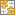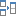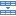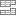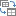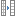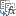# Calculate and highlight 90/60/30 days from or before today in Excel

The tutorial shows how to create a date calculator in Excel exactly for your needs to find a date any N days from or before today, counting all days or only business days.

Are you looking to calculate the expiration date that is exactly 90 days from now? Or you wonder what date is 45 days after today? Or you need to know the date that occurred 60 days before today (counting only business days and all days)?

Whatever your task is, this tutorial will teach you how to make your own date calculator in Excel in under 5 minutes. If you don't have that much time, then you can use our online calculator to find the date that is the specified number of days after or prior to today.

## Date Calculator in Excel Online

Want a quick solution to "what is 90 days from today" or "what is 60 days before today"? Type the number of days in the corresponding cell, press Enter, and you will immediately have all the answers:

Need to calculate 30 days from a given date or determine 60 business days prior to a certain date? Then use this date calculator.

Curious to know what formulas are used to calculate your dates? You will find them all and a lot more in the following examples.

## How to calculate 30/60/90 days from today in Excel

To find a date N days from now, use the TODAY function to return the current date and add the desired number of days to it.

To get a date that occurs exactly 30 days from today:
`=TODAY()+30`

To calculate 60 days from today:
`=TODAY()+60`

What date is 90 days from now? I guess you already know how to get it :)
`=TODAY()+90`

To make a generic today plus N days formula, input the number of days in some cell, say B3, and add that cell to the current date:

`=TODAY()+B3`

Now, your users can type any number in the referenced cell and the formula will recalculate accordingly. As an example, let's find a date that occurs 45 days from today: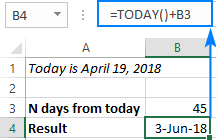#### How this formula works

In its internal representation, Excel stores dates as serial numbers beginning with January 1, 1900, which is the number 1. So, the formula simply adds the two numbers together, the integer representing today's date and the number of days you specify. The TODAY() function is volatile and automatically updates every time the worksheet is opened or recalculated - so when you open the workbook tomorrow, your formula will recalculate for the current day.

At the moment of writing, today's date is April 19, 2018, which is represented by the serial number 43209. To find a date, say, 100 days from now, you actually perform the following calculations:

`=TODAY() + 100`

`= April 19, 2018 + 100`

`= 43209 + 100`

`= 43309`

Convert the serial number 43209 to the Date format, and you'll get July 28, 2018, which is exactly 100 days after today.

## How to get 30/60/90 days before today in Excel

To calculate N days before today, subtract the required number of days from the current date. For example:

90 days before today:
`=TODAY()-90`

60 days prior to today:
`=TODAY()-60`

45 days before today:
`=TODAY()-45`

Or, make a generic today minus N days formula based on a cell reference:

`=TODAY()-B3`

In the screenshot below, we calculate a date that occurred 30 days before today.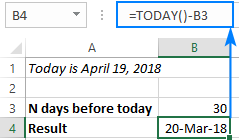## How to calculate N business after/prior to today

As you probably know, Microsoft Excel has a few functions to calculate working days based on a start date as well as between any two dates that you specify.

In the below examples, we will be using the WORKDAY function, which returns a date that occurs a given number of working days ahead of or prior to the start date, excluding weekends (Saturday and Sunday). If your weekends are different, then use the WORKDAY.INTL function that allows custom weekend parameters.

So, to find a date N business days from today, use this generic formula:

WORKDAY(TODAY(), N days)

Here are a few examples:

`=WORKDAY(TODAY(), 10)`

30 working days from now
`=WORKDAY(TODAY(), 30)`

`=WORKDAY(TODAY(), 5)`

To get a date N business days before today, use this formula:

WORKDAY(TODAY(), -N days)

And here are a couple of real-life formulas:

90 business days prior to today
`=WORKDAY(TODAY(), -90)`

15 working days before today
`=WORKDAY(TODAY(), -15)`

To make your formula more flexible, replace the hardcoded number of days with a cell reference, say B3:

`=WORKDAY(TODAY(), B3)`

`=WORKDAY(TODAY(), -B3)`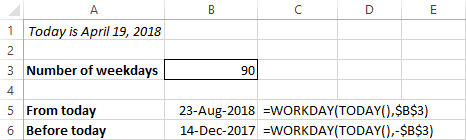In a similar manner, you can add or subtract weekdays to/from a given date, and your Excel date calculator can look like this.

## How to create a date calculator in Excel

Do you remember the Excel Online Date Calculator showcased in the very beginning of this tutorial? Now you know all the formulas and can easily replicate it in your worksheets. You can even craft something more elaborate because the desktop version of Excel provides far more capabilities.

To give you some ideas, let's design our Excel Date Calculator right now.

Overall, there can be 3 choices for calculating dates:

• Based on today's date or specific date
• From or before the specified date
• Count all days or only working days

To provide all these options to our users, we add three Group Box controls (Developer tab > Insert > Form Controls > Group Box) and insert two radio buttons into each group box. Then, you link each group of buttons to a separate cell (right-click the button > Format Control > Control tab > Cell link), which you can hide later. In this example, the linked cells are D5, D9 and D14 (please see the screenshot below).

Optionally, you can enter the following formula in B6 to insert the current date if the Today's date button is selected. It is not actually necessary for our main date calculation formula, just a small courtesy to your users to remind them what date today is:

`=IF(\$D\$5=1, TODAY(), "")`

Finally, insert the following formula in B18 that checks the value in each linked cell and calculates the date based on the user's choices:

`=IF(AND(\$D\$5=1, \$D\$9=1, \$D\$14=1), TODAY()+\$B\$3, IF(AND(\$D\$5=1, \$D\$9=1, \$D\$14=2), WORKDAY(TODAY(),\$B\$3), IF(AND(\$D\$5=1, \$D\$9=2, \$D\$14=1), TODAY()-\$B\$3, IF(AND(\$D\$5=1, \$D\$9=2, \$D\$14=2), WORKDAY(TODAY(),-\$B\$3), IF(AND(\$D\$5=2, \$D\$9=1, \$D\$14=1), \$B\$7+\$B\$3, IF(AND(\$D\$5=2, \$D\$9=1, \$D\$14=2), WORKDAY(\$B\$7, \$B\$3), IF(AND(\$D\$5=2, \$D\$9=2, \$D\$14=1), \$B\$7-\$B\$3, IF(AND(\$D\$5=2, \$D\$9=2, \$D\$14=2), WORKDAY(\$B\$7,-\$B\$3), ""))))))))`

It may look like a monstrous formula at first sight, but if you break it into individual IF statements, you will easily recognize the simple date calculation formulas we've discussed in the previous examples.

And now, you select the desired options, say, 60 days from now, and get the following result: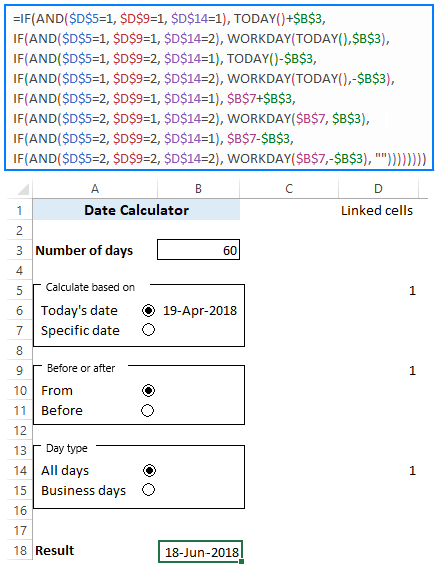To have a closer look at the formula and probably reverse-engineer it for your needs, you are welcome to download our Date Calculator for Excel.

## Special tools to calculate dates based on today

If you are looking for something more professional, you can quickly calculate 90, 60, 45, 30 days from now (or whatever number of days you need) with our Excel tools.

### Date and Time Wizard

If you've had a chance to pay with our Date and Time Wizard at least once, you know that it can instantaneously add or subtract days, weeks, months or years (or any combination of these units) to a certain date as well as calculate the difference between two days. But did you know it can also calculate dates based on today?

As an example, let's find out what date is 120 days from today:

1. Enter the TODAY() formula in some cell, say B1.
2. Select the cell where you want to output the result, B2 in our case.
3. Click the Date & Time Wizard button on the Ablebits Tools tab.
4. On the Add tab, specify how many days you want to add to the source date (120 days in this example).
5. Click the Insert formula button.

That's it!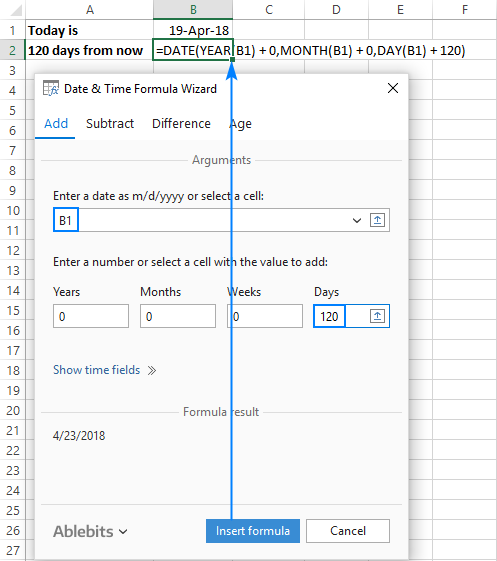As shown in the screenshot above, the formula built by the wizard is different from all the formulas we've dealt with, but it works equally well :)

To get a date that occurred 120 days before today, switch to the Subtract tab, and configure the same parameters. Or, enter the number of days in another cell, and point the wizard to that cell: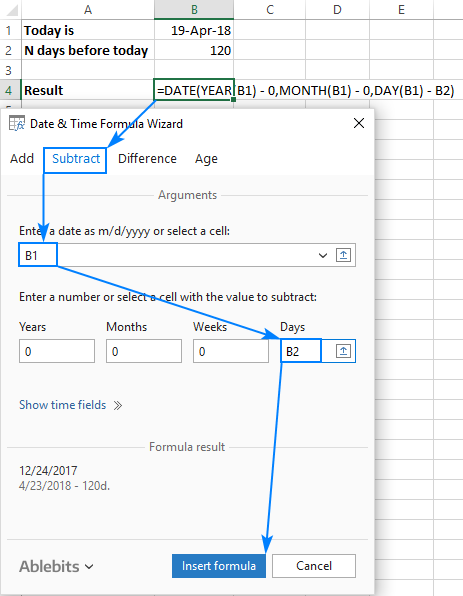As the result, you will get a universal formula that recalculates automatically every time you enter a new number of days in the referenced cell.

### Date Picker for Excel

With our Excel Date Picker, you can not only insert valid dates in your worksheets in a click, but also calculate them!

Unlike the Date and Time Wizard, this tool inserts dates as static values, not formulas.

For example, here's how you can get a date 21 days from today:

1. Click the Date Piker button on the Ablebits Tools tab to enable a drop-down calendar in your Excel.
2. Right-click the cell where you'd like to insert the calculated date and choose Select Date from Calendar from the pop-up menu.
3. The drop-down calendar will show up in your worksheet with the current date highlighted in blue, and you click the calculator button in the upper right corner: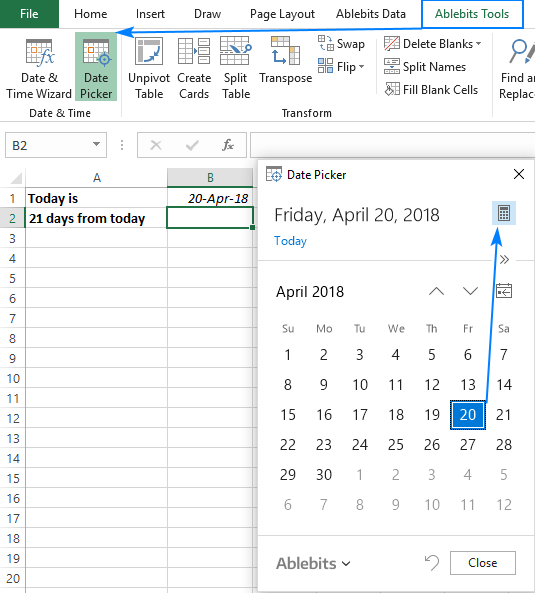4. On the upper pane, click the Day unit and type the number of days to add, 21 in our case. By default, the calculator performs the addition operation (please notice the plus sign in the display pane). If you'd like to subtract days from today, then click the minus sign on the lower pane.
5. Finally, click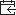to show the calculated date in the calendar. Or, press the Enter key or click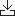to inset the date into a cell: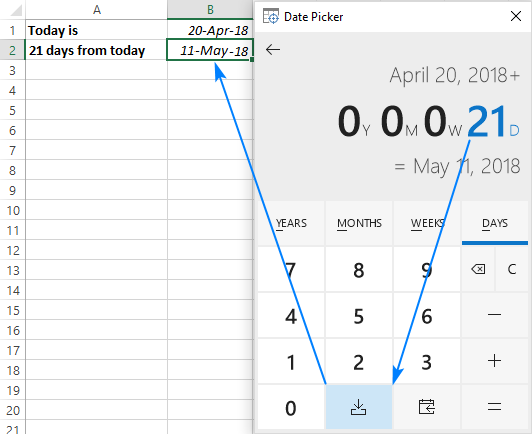## How to highlight dates 30, 60 and 90 days from today

When calculating expiration or due dates, you may want to make the results more visual by color-coding the dates depending on the number of days prior to expiration. This can be done with Excel Conditional Formatting.

As an example, let's make 4 conditional formatting rules based on these formulas:

• Green: more than 90 days from now
`=C2>TODAY()+90`
• Yellow: between 60 and 90 days from today
`=C2>TODAY()+60`
• Amber: between 30 and 60 days from today
`=C2>TODAY()+30`
• Red: less than 30 days from now
`=C2<TODAY()+30`

Where C2 is the topmost expiry date.

Here are the steps to create a formula-based rule:

1. Select all the cells with the expiry dates (B2:B10 in this example).
2. On the Home tab, in the Styles group, click Conditional Formatting > New Rule…
3. In the New Formatting Rule dialog box, select Use a formula to determine which cells to format.
4. In the Format values where this formula is true box, enter your formula.
5. Click Format…, switch to the Fill tab and select the desired color.
6. Click OK two times to close both windows.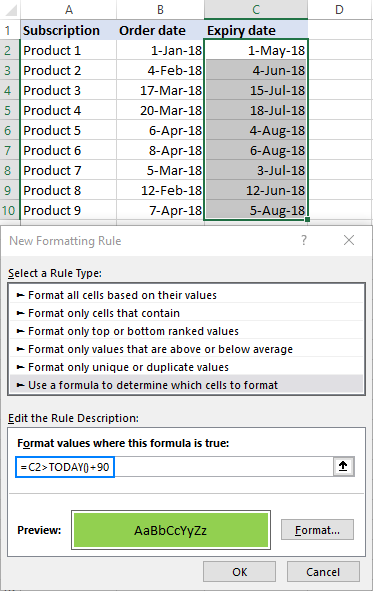Important note! For the color codes to apply correctly, the rules should be sorted exactly in this order: green, yellow, amber, red: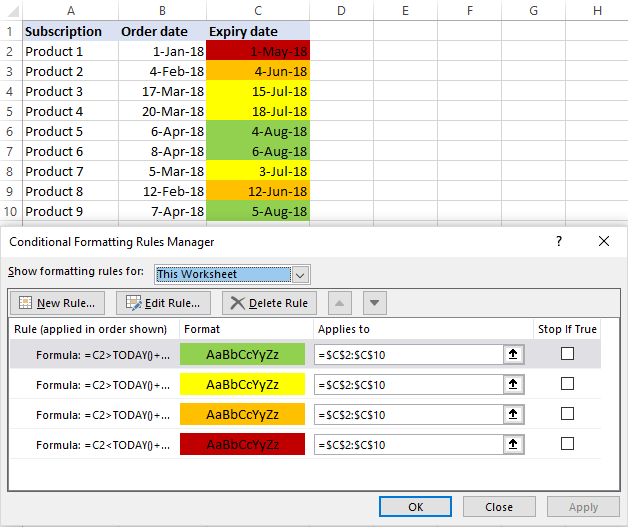If you don't want to bother about the rules order, use the following formulas that define each condition exactly, and arrange the rules as you please:

Green: over 90 days from now:
`=C2>TODAY()+90`

Yellow: between 60 and 90 days from today:
`=AND(C2>=TODAY()+60, C2<=TODAY()+90)`

Amber: between 30 and 60 days from today:
`=AND(C2>=TODAY()+30, C2<TODAY()+60)`

Red: less than 30 days from today:
`=C2<TODAY()+30`

Tip. To include or exclude the boundary values from a certain rule, use the less than (<), less than or equal to (<=), greater than (>), greater than or equal to (<=) operators as you see fit.

In a similar manner, you can highlight past dates that occurred 30, 60 or 90 days ago from today.

• Red: more than 90 days before today:
`=B2<TODAY()-90`
• Amber: between 90 and 60 days before today:
`=AND(B2>=TODAY()-90, B2<=TODAY()-60)`
• Yellow: between 60 and 30 days before today:
`=AND(B2>TODAY()-60, B2<=TODAY()-30)`
• Green: less than 30 days before today:
`=B2>TODAY()-30`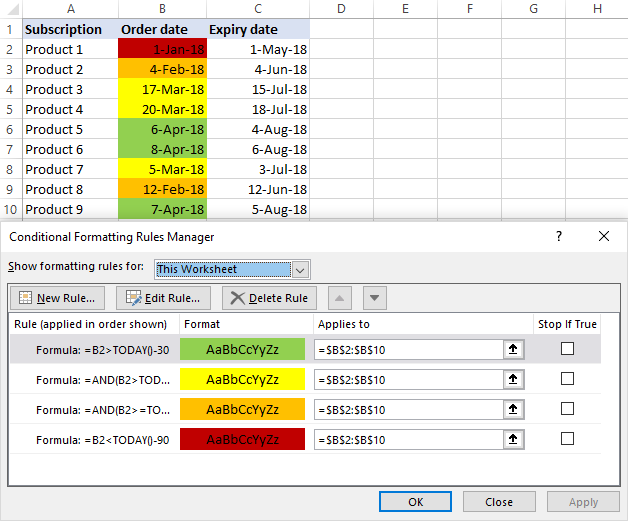More examples of conditional formatting for dates can be found here: How to conditionally format dates and time in Excel.

That's how you calculate dates that are 90, 60, 30 or n days from/before today in Excel. To have a close look at the formulas and conditional formatting rules discussed in this tutorial, I invite you to download our sample workbook below. Thank you for reading and hope to see you on our blog next week!

Calculate Dates in Excel - examples (.xlsx file)

### 28 responses to "Calculate and highlight 90/60/30 days from or before today in Excel"

1.Sudheesh says:

Hi Svetlana,

If total 85000 then rates as per below slab
0 to 40000 (40000 x 0.50)
40001 to 80000 (40000 x 0.45)
(balance 5000 x 0.425
how to get the formula in one cell (total), please advise the formula.
Thanks.

•Mikey says:

nested if will do the trick:

=IF(A1<=40000,A1*0.5,IF(A1 19500
45000 --> 22250
85000 --> 40125

•Mikey says:

code got eaten. if you see \$lt; below, that is less than symbol.

=IF(A1<=40000,A1*0.5,IF(A1<=80000,SUM(20000,(A1-40000)*0.45),SUM(38000,(A1-80000)*0.425)))

2.SHYNI says:

ARRIVAL DATE : 25.07.2018

WHAT IS THE FORMULA FOR FINDING 90 DAYS FROM THE ARRIVAL DATE

3.Doug says:

SHYNI:
Excel will calculate future dates using this formula:
=DATE(YEAR(H4)+2,MONTH(H4)+5,DAY(H4)+3)
This will return 12/28/2020 from your sample date.
To return 10/23/18 or 90 days from your sample date use this: =DATE(YEAR(H4),MONTH(H4),DAY(H4)+90)
You might be tempted to enter a "+3" in the month spot, but if you do Excel will return 10/25/18 from your sample date. So, three months is not the same thing as 90 days in Excel date calculations.
Be sure the date you enter is in a format Excel recognizes as a date and that the cells you use in these calculations are formatted as dates.
Play around with this formula and you'll quickly see how to use it.

4.Carlos A Ibarra says:

Hi, How can add amounts based on 30+ days from origination?

Date Amount
Draw 1 6/1/18 500.00
Draw 2 6/15/18 500.00
Draw 3 7/1/18 500.00
Draw 4 7/15/18 500.00

Since June 1 and 15 have more than 30 days from today 7/23/18 the result should be 1,000.00

I have tried many different ways with no success, any help will be appreciated.

Thank you,
Carlos

5.Tr says:

Hi,

How would I use data validation to calculate a start and end date that must fall -90 days from todays date and be in the current year. The date has to be in current year even if the prior year falls within the -90 back from today whatever today will be?

regards

6.Ugi says:

Hi, How can add amounts based on 30+ days from origination?

7.Sagar says:

I need Employee business before 30 of training date & after 30 days of training date.

i.e. If my Employee training date is 15th Oct, so i need before 30 days of business (14th Sep to 15th oct)& after 30 days of business (16th Oct to 15th Nov)

Which formula I can use for above query.

8.Samuel says:

I want to populate my timesheet with the correct date for each day that updates automatically, picks the month in words automatically in a different cell.

9.Cristal De La Torre says:

Hello,
I am trying to have column F to turn red if it is past 30 days of the date in column E which will have a date or NO. I've red several forums need help :(
thank you!
Cristal

10.Giri prasad says:

Hi
I need calculate sum if bill age b/w 1-30, 30-60, 60-90 etc...
Net pay Date Ageing
79500 18-Sep-11 2699.00
54500 20-Jan-19 18.00
14500 14-Sep-18 146.00
13500 10-Dec-18 59.00
64500 16-Jan-19 22.00
9000 10-Jan-19 28.00
24500 10-Nov-18 89.00
22500 10-Nov-18 89.00
21500 10-Nov-18 89.00
74500 10-Jul-18 212.00
34500 10-Jul-18 212.00
34500 10-Jul-18 212.00

Below 30 Below 30-60 Below 60-90

Hope u can help reg this
Thanks & Reg

11.Mike says:

I have a set of 4 dates set at three months apart through the year, I want them to turn Amber when the (TODAY) date is in the same month, and then turn RED if the date passes.
Is this achievable?

Mike D

12.Tracy says:

How do I highlight the below in red 30 days before the dates shown?
5 yearly
"Electrical
Test"
30.04.23
01.09.19
01.09.19
01.08.19
01.08.19
24.01.21
01.09.21
01.07.22
28.03.23
01.12.22

13.Rosalina says:

Hello,
Does anyone know how I can use Conditionnal Formatting in Google Sheets to auto highlight the current date ?
Lets say I enter a deadline as 22/03/2019 on that specific date I would like the cell to turn into a specific colour.

14.Vishak says:

I have a bug open for 100 days and I want to add it in the bucket( 30-60days), (60-90 days)
How to achieve it in google sheets

15.Archana says:

Hi Team,

I need a formual to calculate the cooling period which is of 90 days. below is the sample given Please help me for same.

Date of CV Received Validity Date Days remaining
28-Mar-19 27-Jun-19 90 days
Regards,
Archana

16.Evan Landry says:

SO after you do the conditional formatting for the dates, I want to be able to track how many dates I have between 1-30 days, meaning +1 day of today's date to 30 days, 31-60 days and 61-90 days after the calculations have been done as a roll up. How do I do that?

•Svetlana Cheusheva (Ablebits.com Team) says:

Hi Evan,

This can be done by using the COUNTIFS function.

For example, to count dates between 1-30 days from today, use this formula:
=COUNTIFS(C2:C10, ">="&TODAY()+1, C2:C10, "<="&TODAY()+30)

Where C2:C10 are the dates to be counted.

17.Nick says:

Hello, I'm trying to create a spreadsheet for a unit tracker while i'm deployed overseas, and I would like to be able to have a cell highlight in Green, Yellow, and Red when it is within 90, 60, and 30 days from a specified date.

For example, in H14 i have the date 30 DEC 20. I would like G14 to highlight Green when it is Nov 30 to Dec 30, Yellow when it is Oct 30 to Nov 30, and Red when it is Sep 30 to Oct 30

If it is not possible, red when it is 90 days from the specified date would be great as well.

I'm relatively new and inexperienced when it comes to Excell, but I am slowly learning more.

Thank you very much!

•Alexander Trifuntov (Ablebits.com Team) says:

Hello Nick!
To select cells 30 days before a specific date (A1), use the formula for conditional formatting

=IF(AND((\$A\$1-\$B2)<30,(\$B2<\$A\$1)),TRUE,FALSE)

I hope this will help

18.ગીરીશ says:

I want to calculate day 1 Jan 2020 to 31 Jan 2020 but I can't get answer 31 why? Which formula to possible it. I want to calculate starting date and end date both
Plz give me excel formula

•Alexander Trifuntov (Ablebits.com Team) says:

Hello!
Excel considers 31 Jan 2020 as 31-Jan-2020 00:00:00. Therefore, from 01-Jan-2020 00:00:00 to 31-Jan-2020 00:00:00 - 30 days.

19.Liiam says:

Hi i am hoping someone can help.
I basically have a matrix thats needs to calculate and highlight dates.

So for example if a due date is 28 days from today - green
If it is 9 days from today - orange
if the date is the same date as today or past - red

but then another trick is that i dont want the box to remain red once the date is past if a completion date is entered in another cell. can anyone help with this?

20.tammy friedman says:

I need the formula for 30 days past a certain date. Example today is the 16th of November. I need the next cell to be 30 days past todays date. December 30 will be 30 days.

•Alexander Trifuntov (Ablebits.com Team) says:

Hello!
Based on your description, it is hard to completely understand your task. However, I’ll try to guess and offer you the following formula:

=A1+30

Why "December 30 will be 30 days"?

Ultimate Suite for Microsoft Excel